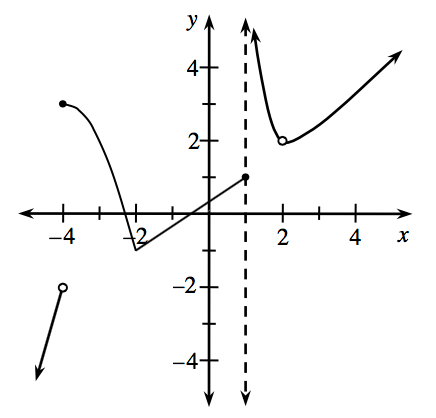### Home > APCALC > Chapter 8 > Lesson 8.1.1 > Problem8-10

8-10.

Use the graph of $y = g(x)$ at right to answer the following questions. Provide reasons for each value found.

1. For what values of $x$ is $g(x)$ undefined?

Are there any $x$-values for which there is no existing $y$-value?

2. For what values of $x$ is $g\left(x\right)$ discontinuous?

There are three conditions of continuity.
If any of these conditions are violated, then the function is NOT continuous at the value of $x$.

$1. \ \ \lim\limits_{x\rightarrow a}g(x)\text{ exists.} \ \ \ \text{This means that }\lim\limits_{x\rightarrow a^{-}}g(x)=\lim\limits_{x\rightarrow a^{+}}g(x) \text{ and that limit is finite.}$

2. $f(a)$ exists. This means that $f(a)$ is finite.

$3. \ \ \ \lim\limits_{x\rightarrow a}g(x)=f(a)$

$y = g(x)$ has exactly three points in which it is discontinuous. Where are they?

3. For what values of $x$ is $g(x)$ non-differentiable?

A function is NOT differentiable where it is not continuous and/or where the derivative is not continuous. Examples of points of non-differentiability include cusps, endpoints, jumps, holes, and vertical tangents.